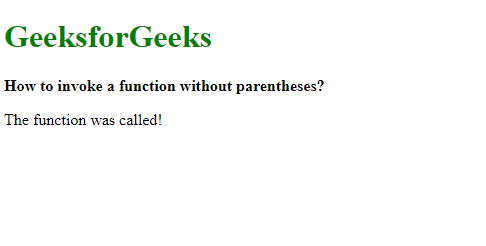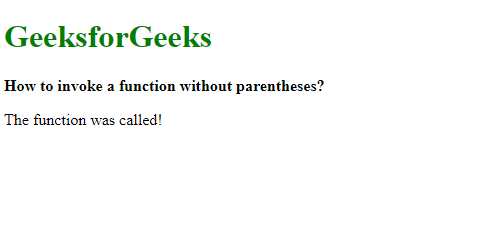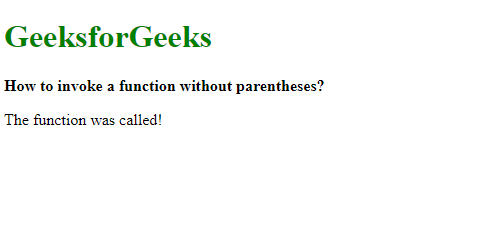# How to invoke a function without parentheses using JavaScript ?

• Last Updated : 31 Dec, 2019

Method 1: Using the new operator: The new operator is used to create an instance of an object which has a constructor function. This constructor function can be used to write our own function and then be invoked by the new operator. The parentheses are optional when using this operator, hence the function would be invoked even without them.

Syntax:

`new exampleFunction`

Example:

 ` ` `<``html``> ` `<``head``> ` `    ``<``title``> ` `        ``How to invoke a function ` `        ``without parentheses? ` `    `` ` ` ` `<``body``> ` `    ``<``h1` `style``=``"color: green"``> ` `        ``GeeksforGeeks ` `    `` ` `    ``<``b``> ` `        ``How to invoke a function ` `        ``without parentheses? ` `    `` ` `    ``<``p` `class``=``"output"``>  ` `     `  `    ``<``script` `type``=``"text/javascript"``> ` `        ``function exampleFunction() { ` `            ``document.querySelector(".output").textContent ` `                  ``= "The function was called!"; ` `        ``} ` ` `  `        ``// Using the new operator ` `        ``new exampleFunction; ` `    `` ` ` ` ` `

Output:Method 2: Using tagged template literals: Tagged template literals are a use case of template literals that was introduced in JavaScript ES6. It allows the use of functions directly when using template literals. It can be used with parameters bypassing them in the literals. To invoke a function, its name is written along with back-ticks (` `) to denote it as tagged template literals.

Syntax:

`exampleFunction```

Example:

 ` ` `<``html``> ` `<``head``> ` `    ``<``title``> ` `        ``How to invoke a function ` `        ``without parentheses? ` `    `` ` ` ` `<``body``> ` `    ``<``h1` `style``=``"color: green"``> ` `        ``GeeksforGeeks ` `    `` ` `    ``<``b``> ` `        ``How to invoke a function ` `        ``without parentheses? ` `    `` ` `    ``<``p` `class``=``"output"``>  ` `     `  `    ``<``script` `type``=``"text/javascript"``> ` `        ``function exampleFunction() { ` `            ``document.querySelector(".output").textContent ` `                    ``= "The function was called!"; ` `        ``} ` ` `  `        ``// Using tagged template literals ` `        ``exampleFunction``; ` `    `` ` ` ` ` `

Output:Method 3: Modifying the toString() method: The toString() method is invoked whenever a string conversion is necessary. An object can be created which has the toString() method modified to call the required function. This object can be used to call toString() by performing a concatenation with an empty string. It will force the toString() method to be called which will in turn, invoke the specified function.

Syntax:

 `let tempObj = { ` `    ``toString: exampleFunction ` `} ` ` `  `''` `+ tempObj; `

Example:

 ` ` `<``html``> ` `<``head``> ` `    ``<``title``> ` `        ``How to invoke a function ` `        ``without parentheses? ` `    `` ` ` ` `<``body``> ` `    ``<``h1` `style``=``"color: green"``> ` `        ``GeeksforGeeks ` `    `` ` `    ``<``b``> ` `        ``How to invoke a function ` `        ``without parentheses? ` `    `` ` `    ``<``p` `class``=``"output"``>  ` `     `  `    ``<``script` `type``=``"text/javascript"``> ` `        ``function exampleFunction() { ` `            ``document.querySelector(".output").textContent ` `                   ``= "The function was called!"; ` `        ``} ` ` `  `        ``let tempObj = { ` `          ``toString: exampleFunction ` `        ``} ` ` `  `        ``// Forcing the modified  ` `        ``// toString function to be invoked ` `        ``'' + tempObj; ` `    `` ` ` ` ` `

Output:My Personal Notes arrow_drop_up
Recommended Articles
Page :